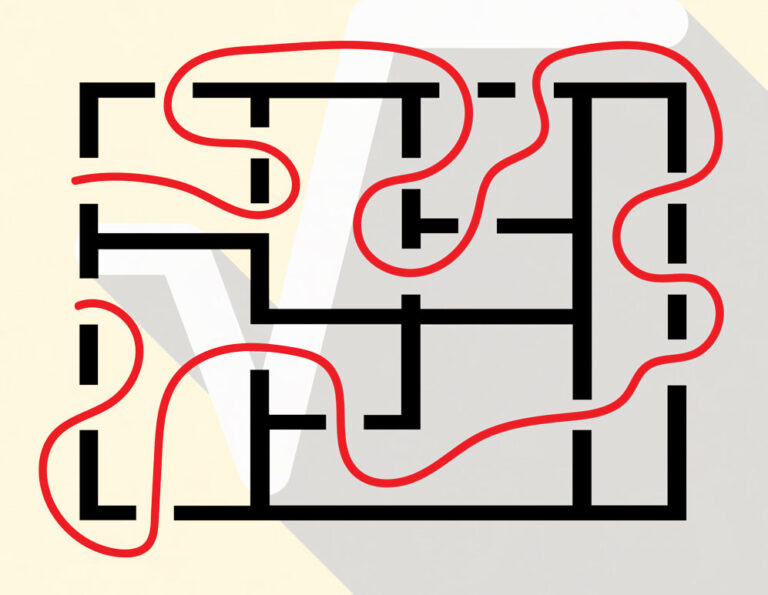# Getting to the Root of ThingsCan you simplify the following expression into a rational number? If you can, then do it.

√(1+1000000√(1+1000001√(1+1000002√(1+1000003√(1+1000004√(1+…)) ) ) ) )